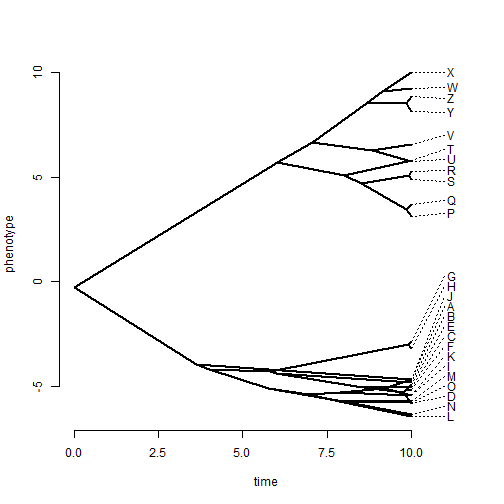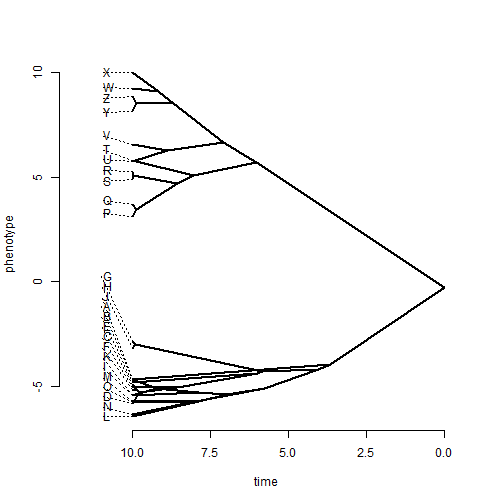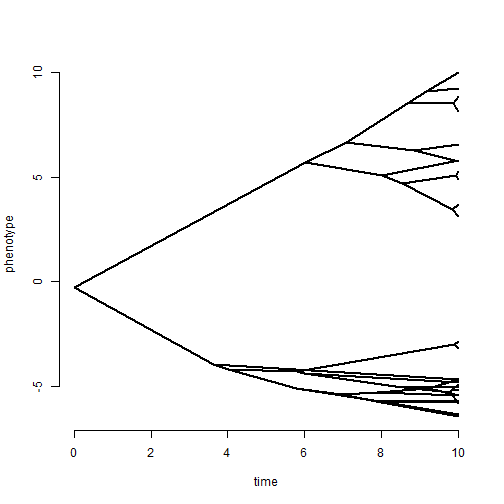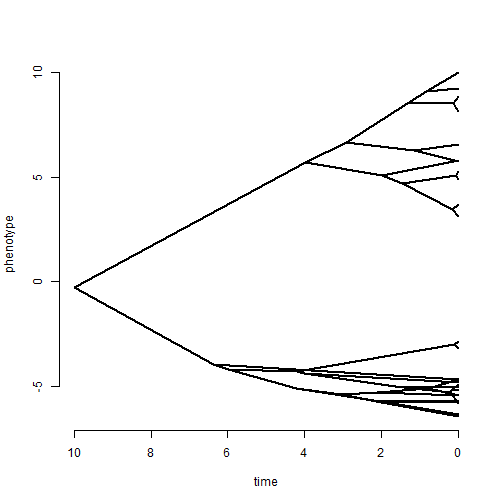## Friday, December 4, 2015

### Flipping the axis of a plotted traitgram

I received an inquiry the other day about how to plot a traitgram (that is a projection of the tree into a space defined by phenotype on one axis, and time on the other) such that the deepest node in the tree had time equal to the total tree height, and the x-axis runs from right to left.

Well, although the axis can be easily flipped in the function `phenogram` (by merely setting `xlim > xlim`, this also has the effect of flipping the plotted traitgram. For instance:

``````library(phytools)
phenogram(tree,x) ## default settings
````````````phenogram(tree,x,xlim=par()\$usr[2:1]) ## flipped
``````Of course, we see that the labels are offset in the wrong direction when we do this.

Now, it turns out that it is not too difficult to create this type of projection using functions in the phytools package, so if we want to generate the visual I described above, we should be able to as follows. (BTW, the algorithms to do generate this & many other visualizations of phylogenies are described in my chapter in the book Modern Phylogenetic Comparative Methods and Their Application in Evolutionary Biology, edited by László Garamszegi.)

First, in the standard direction:

``````xa<-c(x,fastAnc(tree,x))
H<-nodeHeights(tree)
Y<-matrix(xa[tree\$edge],nrow(tree\$edge),2)
plot.new()
plot.window(xlim=range(H),ylim=range(Y))
axis(1)
axis(2)
for(i in 1:nrow(tree\$edge)) lines(H[i,],Y[i,],lwd=2)
title(xlab="time",ylab="phenotype")
``````Then, with the axis running backwards from 0 to 10, but the traitgram projection still pointing from left to right:

``````xa<-c(x,fastAnc(tree,x))
H<-nodeHeights(tree)
H<-max(H)-H
Y<-matrix(xa[tree\$edge],nrow(tree\$edge),2)
plot.new()
plot.window(xlim=range(H)[2:1],ylim=range(Y))
axis(1)
axis(2)
for(i in 1:nrow(tree\$edge)) lines(H[i,],Y[i,],lwd=2)
title(xlab="time",ylab="phenotype")
``````Simple as that. We can, for instance, overlay this in any way we want on existing plots & other such things.

That's it!

The data used to produce this example were simulated using the following code:

``````tree<-pbtree(n=26,tip.label=LETTERS,scale=10)
x<-fastBM(tree)
``````

#### 1 comment:

1.Hi Liam. I have a couple of questions about plotting the phenogram:
1. How can you restore the tips in the last example (reversed axis, tips to the right). It is possible to reverse the x-axis from the arguments when calculating the phenogram?
2. How can you add a detailed geological scale (75 ma) to the phenogram?
Thanks!

2. It is possible to

Note: due to the very large amount of spam, all comments are now automatically submitted for moderation.